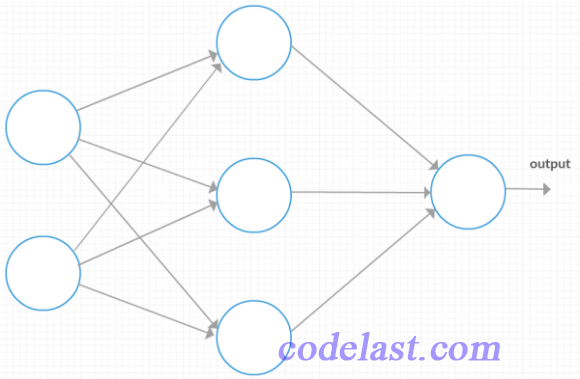# [原创] 《Neural Networks and Deep Learning》读书笔记：最简单的识别MNIST的神经网络程序(1)

Neural Networks and Deep Learning》一书的中文译名是《神经网络与深度学习》，书如其名，不需要解释也知道它是讲什么的，这是本入门级的好书。

『1』原文及程序

class Network(object):

def __init__(self, sizes):
self.num_layers = len(sizes)
self.sizes = sizes
self.biases = [np.random.randn(y, 1) for y in sizes[1:]]
self.weights = [np.random.randn(y, x)
for x, y in zip(sizes[:-1], sizes[1:])]

net = Network([2, 3, 1])
Network对象的偏差和权重都是被随机初始化的，使用Numpy的np.random.randn函数来生成均值为0，标准差为1的高斯分布。随机初始化给了我们的随机梯度下降算法一个起点。在后面的章节中我们将会发现更好的初始化权重和偏差的方法，但是现在将采用随机初始化。注意Network初始化代码假设第一层神经元是一个输入层，并对这些神经元不设置任何偏差，因为偏差仅在之后的层中使用。

『2』程序解读

self.biases = [np.random.randn(y, 1) for y in sizes[1:]]

a = [i for i in range(3)]
print(a)

[0, 1, 2]

a = [5, 6, 8]
print(a[1:])

[6, 8]

np 是这个Python程序 import 进来的Numpy库的缩写：

import numpy as np

randn() 是Numpy这个库中，用于生成标准正态分布数据的一个函数。其实 randn(3, 1) 生成的是一个3x1的随机矩阵，我们可以在Python命令行中直接试验一下：

import numpy as np

np.random.randn(3, 1)

array([[ 1.33160979],
[ 0.66314905],
[ 0.27303603]])

self.weights = [np.random.randn(y, x) for x, y in zip(sizes[:-1], sizes[1:])]

for x, y in zip(sizes[:-1], sizes[1:])

zip([3, 4], [5, 9])

[(3, 5), (4, 9)]

for x, y in zip([3, 4], [5, 9])

biases：3x1 的矩阵
biases：1x1 的矩阵
weights：3x2 的矩阵
weights：1x3 的矩阵➤➤ 版权声明 ➤➤### 《[原创] 《Neural Networks and Deep Learning》读书笔记：最简单的识别MNIST的神经网络程序(1)》有4条评论

1. 感谢如此细致的讲解

回复
2. 求全系列的《Neural Networks and Deep Learning》读书笔记的更新，讲的实在太好了。

回复
3. 太棒了，什么时候更新

回复